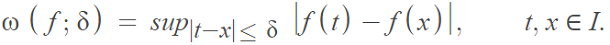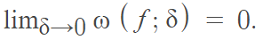# Modulus of Continuity

The modulus of continuity, introduced by Lebesgue in 1910, is a positive value that characterizes how continuous a given function is. More specifically, by the (ε, δ) definition of continuity, real functions are continuous if they have a modulus of continuity at each point x in the domain . It can be used to investigate convergence or to estimate the order of a function’s approximation by polynomials or other methods.

## Formal Definition of the Modulus of Continuity

The term has a few different definitions, some of which are more general than others. One of the more general definitions :

A modulus of continuity is a continuous, increasing function ω: [0, ∞) → [0, ∞) with ω(0) = 0.

Other definitions may have restrictions on the domain, exclude the requirement of a continuous function (in which case the continuity is replaced with continuity at zero), or have different intervals. For example, the domain can also be defined as: [0, 1] → [0, ω) and the intervals can be:

• [a, b],
• (a, b),
• (-∞, ∞),
• [a, b).

While ω is the most common notation, other notation is often used. For example, Agbor  defines the modulus of continuity in an even more general sense, as a function σ “…defined on the non-negative reals, which tends to zero at the origin.”

## Formula for Modulus of Continuity

The modulus of continuity ω is defined by :Where:

• f = a uniformly continuous function,
• sup = supremum,
• I = a finite or infinite interval.

The modulus of continuity depends on δ, f, and the interval [a, b]. So ω(δ) is short for ω(f; [a, b]; δ).

The function ω has the propertyIn other words, a function f is uniformly continuous on a specific interval only if the above limit holds. This useful property means that the modulus of continuity can be used to estimate the order of a function’s approximation by entire functions, polynomials, or sequences.

Another way to look at it :
Assume that on an interval I there is a function f. Choose a positive number δ and consider all pairs (x, y) which belong in the interval and satisfy the inequality:
|x – y| < δ.
The upper limit (supremum) of the function values |f(x) – f(y)|, which can also be infinite, is the modulus of continuity. It shows the extent to which two function values can differ from each other, if we know that they don’t differ by more than δ.

## References

 Weihrauch, K. (2000). Computable Analysis: An Introduction. Springer.
 Breneis, S. (2020). On variation functions and their moduli of continuity. Journal of Mathematical Analysis and Applications, Volume 491, Issue 2, 15 November.
 Agbor, A. (2012). Topics in analysis – Modulus of continuity of mappings between Euclidean spaces – Toeplitz operators on the Bergman spaces of the unit
ball in ℂN. Retrieved May 2, 2021 from: https://www2.math.su.se/reports/2012/2/2012-2.pdf
 Gadjiev, A. & Aral, A. (2007). The estimates of approximation by using a new type of weighted modulus of continuity. Computers and Mathematics with Applications 54 127–135. Retrieved May 5, 2021 from: https://core.ac.uk/download/pdf/82365827.pdf
 Natanson, I. (1961). Constructive Theory of Functions, Volumes 1-2. University of Michigan Library.

CITE THIS AS:
Stephanie Glen. "Modulus of Continuity" From StatisticsHowTo.com: Elementary Statistics for the rest of us! https://www.statisticshowto.com/modulus-of-continuity/